﻿ Hubble's quantum law. Cosmological redshift. Hubble constant. Big Bang theory. Doppler effect. Aging of the light. Tired.
 Sergey B. Alemanov  http://alemanow.narod.ru  alemanow@mail.ru Hubble's quantum law  vn = nH0 (Quantum law of the cosmological redshift)   A report in the Moscow State University (13.03.2013, 18.10.2013), Prokhorov General Physics Institute (29.01.2014), Moscow Aviation Institute (29.05.2015), RUDN University (28.04.2016). Published in the «Engineering Physics» (No. 3, 2014).

## Hubble constant is the quantum amount by which a photon's frequency decreases in one period of oscillation.

Hubble's law, as presented in quantum form, allows exact calculation of the cosmological redshift for galaxies at any distance. For example, the distance to galaxies, as calculated on the basis of the contemporary method of "standard candles" (Nobel Prize 2011) mismatches the one calculated on the basis of the Doppler effect (the Big Bang theory), while it is fully consistent with Hubble's quantum law.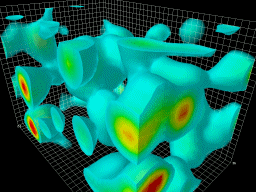Vacuum fluctuations.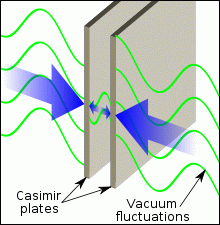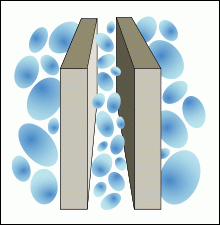The pressure of the vacuum fluctuations on the parallel plates. The static Casimir effect.

When the cosmological redshift was discovered, it was unknown that vacuum fluctuations existed and respectively, that the distribution of the electromagnetic waves is always accompanied by the energy dissipation due to the transformation of this energy into the inner energy of the vacuum. Therefore the only - as it then seemed - possible explanation was put forward: the Doppler effect. But not all scientists agreed to it, and the disputes continued. Some thought it to be connected with the Doppler effect, while others - including Edwin Hubble - thought that "the aging of light" ("tired light") happens, because ideal waves without dissipation simply do not exist.

«Edwin Hubble, who discovered the redshift, let us see that it is connected not to the Doppler effect, but to the effect of the increase of the light's wavelength as light moves.»
http://ritz-btr.narod.ru/privid.html (In Russian)

Oscillation of the waves is the transformation of one energy form into another and back, and any transformation of energy is inevitably accompanied by a partial loss; the energy of the waves is being dissipated, which converts into the inner energy of the medium expressed in form of fluctuations. The waves' propagation in the physical vacuum is no exception, as, according to quantum physics, a vacuum is not a void. There are fluctuations in it as in any environment. They are called zero-point vibrations of the electromagnetic field. It is the energy dissipation of electromagnetic waves that creates a cosmological redshift, not the Doppler effect caused by the acceleration of the galaxies by supernatural dark energy.

«Hubble's Law is an empirical law connecting in a linear fashion the redshift of the galaxies and distance to them: cz = H0r, where z - the redshift of the galaxy, r - the distance to it, H0 - coefficient of proportionality, called Hubble constant, c - velocity of the light.»
http://ru.wikipedia.org/wiki/Закон_Хаббла (In Russian)
« H0 = 2.4·10-18 s-1(Hz) »
http://en.wikipedia.org/wiki/Hubble_constant
«Using the Hubble's classical law z = H0r/c to determine distances to galaxies ...»
http://www.astrogalaxy.ru/858.html (In Russian)

Hubble's law is associated with the redshift of the electromagnetic quants, that is why, in accordance with quantum physics, it must be presented not only in classical but also in quantum form. For this purpose into the formula of redshift dependency on time z = H0r/c = H0t the time of one oscillation period should be added T = 1/v, where v - the frequency of the photon, H0 - Hubble constant 2.4·10-18 с-1(Hz). We receive the redshift per one oscillation period ZT = H0T = H0/v and vZT = H0 - the amount by which photon's frequency decreases during one oscillation period (vz = v0 - v), and respectively, vn = nH0 - a quantum form of the Hubble's law, where n is the number of oscillations performed during certain traveled distances n = t/T = r/λ = rv/c, vn = v0 - v, λ - wave length λ = c/v. That is to say, Hubble's constant is the quantum amount by which photon's frequency decreases in one period of oscillation irrespective of the wavelength, and to determine by how much the frequency of the photon has decreased, the Hubble constant must be multiplied by the number of oscillations performed: vn = nH0 - formula for the photon's frequency redshift. For example, a photon with a frequency of 6·1014 Hz (λ = 5·10-7 m), having travelled 40 megaparsec (r = 1.234·1024 m), will perform a number of oscillations n = r/λ = rv/c = 2.47·1030. This would decrease its frequency by

vn = nH0 = 2.47·1030 × 2.4·10-18 Hz = 5.9·1012 Hz,

and the redshift would be

z = vn/v = 5.9·1012 Hz / 6·1014 Hz = 0.01
( z = vn/v = nH0/v = rvH0/cv = H0r/c = H0t = 0.01 ).

At short distances - a full match with the Hubble's classical law

z = H0r/c = (2.4·10-18 Hz × 1.234·1024 m) / 3·108 m/s = 0.01.

The dissipation of the photon energy per one oscillation: ET = hH0 = 1.6·10-51 J - the quantum of energy dissipation of the photon (hH0/c2 = 1.8·10-68 kg - the quantum of mass), where h - Planck constant. The maximum number of vibrations, which a photon is capable of doing during its lifetime: N = E/ET = hv/hH0 = v/H0, where E - is the photon energy.

After putting Hubble's law into its quantum form vn = nH0 it becomes apparent that the cosmological redshift of the photon's frequency has a quantum nature and depends only upon the number of oscillations per traveled distance. That is to say, this is a quantum effect, where the wave's energy is dissipated. There is no dependency on the number of oscillations in the case of the Doppler effect. If the frequency decreases by the Hubble constant with each new period, then such process presents wave energy dissipation and not the Doppler effect.

Hubble's quantum law (quantum law of the cosmological redshift) states that a photon's frequency decreases with each new wave oscillation by a quantum amount equal to the Hubble constant, vn = nH0,which is being observed as a redshift. Due to the fact that the period of oscillation constantly increases, frequency decreases with time t according to the law of exponent v(t) = v0e-H0t, and the length of the wave increases λ(t) = λ0eH0t.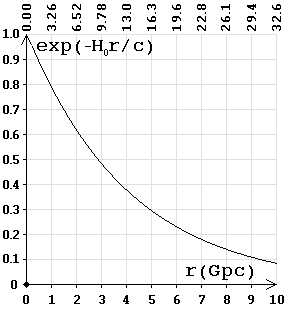Frequency (energy) dependency on the distance. On top of the chart is the time (billion years).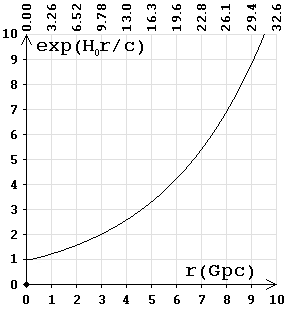Wavelength dependency on the distance. On top of the chart is the time (billion years)

The graph shows that over 9.3 billion years (2.9 gigaparsecs) the frequency is reduced by half. The frequency of the photon is directly proportional to the energy; respectively, E(t) = E0e-H0t - is the formula for photon attenuation, where the Hubble constant represents the parameter of the attenuation of electromagnetic oscillations. This provides total agreement with the exponential law of damped oscillations.

«DAMPED OSCILLATIONS, the natural oscillations, the amplitude A of which decreases with time t according to the law of the exponential A(t) = A0e-at (a - parameter of the attenuation) due to the energy dissipation because of the viscous friction forces for damping mechanical vibrations and the ohmic resistance for electromagnetic damped oscillations.»

The study of the work of Nobel Prize winners in 2011 on the distant type Ia supernovae, attested that the observed redshift of the frequency for both the near and distant galaxies coincides with v(t) = v0e-H0t (formula of dependency of the photon frequency on time). The expression reflects an exponential law of damped oscillations, where H0 - parameter of the attenuation, which is the amount by which the frequency is reduced in one period of oscillation. That is, if the oscillation period t is t = 1/v0, then, regardless of the frequency it is always v(t) = v0 - H0. For example, for distant galaxies at 3.3 Gpc (t = 10.76 billion years = 3.43·1017 seconds), the observed cosmological redshift would be

z = (v0 - v(t)) / v(t) = v0/v(t) - 1 = 1/e-H0t - 1 = eH0t - 1 = 1.3,

rather than z = 2, as assumed in the theory of Universe's expansion, hence the brightness of the supernova is lower.

«With displacements z = 2 ... speed v = 0.8 c ... the distance by the redshift - is r = 3.3 Gpc (the Doppler effect)»
http://ru.wikipedia.org/wiki/Космологическое_красное_смещение (In Russian)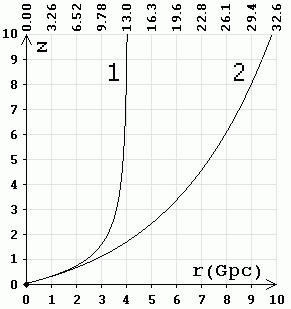1 - according to the theory of the Universe expansion (the Doppler effect). 2 - according to the Hubble quantum law (by the method of "standard candles"). On top of the chart is the time (billion years).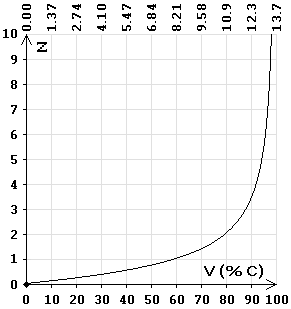Speed dependence on the redshift by the Doppler effect (as percentage of the light speed). On top of the chart is the time (billion years).

«In the works of the Nobel Prize winners in 2011 it was discovered that the far away galaxies, the distance to which was calculated by the Hubble's law, the type Ia supernova's have a brightness lower than the one they were supposed to have. In other words, the distance to these galaxies calculated by the method of the "standard candles" turns out to be larger than the distance calculated on the basis of the previously set value of the Hubble parameter. It was concluded that the Universe is not just expanding, but that it is expanding with acceleration!»
http://hepd.pnpi.spb.ru/ioc/ioc/line%209-10-2011/n5.htm (In Russian)

«Thus, by studying distant from Earth supernovae's, the winners found that those at least a quarter fainter than it had been predicted by the theory, that means the stars are farther than determined from the calculations.»
http://lebed.com/2011/art5913.htm (In Russian)

So studying distant supernovae's, the winners have found that the distance calculated by the Doppler Effect does not correspond with the actual distance to the stars. As of today Ia supernovae's are observed up to z = 2. At z = 2, the distance calculated by the Doppler effect, is 10.8 billion light-years (3.3 Gpc), and by the method of "standard candles" 14.8 billion light-years (4.5 Gpc) redshift increases exponentially z = eH0t - 1. Hence usually a wrong conclusion is made that the Universe is expanding exponentially.

«... the scale factor of the Universe behaves asymptotically like this: a(t) ~ eH0t - the Universe will expand exponentially, and this has not really been expected earlier. That is, it is the accelerated expansion of the Universe, but before that, according to the standard theory, it appeared that the Universe should expand with the slowdown»
Lecture A.D.Linde. http://elementy.ru/lib/430484 (In Russian)

According to the method of "standard candles" the following is established: z + 1 = a0/a(t) = eH0t and z = eH0t - 1, that is the astronomical observations attest that the redshift is subject to exponential law of damped oscillations.

«... a(t) ~ eH0t ... z + 1 = a0/a(t), where a(t) - cosmological scale factor at time t, and a0 - its value at the moment.»
http://www1.jinr.ru/Pepan/2012-v43/v-43-3/01_dol.pdf (In Russian)

During the studies of distant galaxies it was discovered that cosmological redshift occurs according to the law of the exponentially damped oscillations, in accordance with the Hubble's quantum law, not with the Doppler effect, then - for the sake of the salvation of the Big Bang theory and against all laws of physics - a 'miraculous' explanation was invented: the accelerated expansion of the Universe due to dark energy (force). But in this case, the speed of the young galaxies must be less than the speed of the old ones, such as our galaxy, and, therefore, our time should slow down more. Because of this, the observed (by us) duration of the outbreaks of the distant supernovae's should decrease, and not increase.

«This way the galaxies, spectrum of which had the observed double increase of the wavelength of light, had their supernova explosions extended also by two times: from two weeks to four weeks.»
http://www.ritz-btr.narod.ru/compress.html (In Russian)

For each shift, red or purple (due to the Doppler effect, or under the influence of gravity, etc.), always changes not only the wavelength, but also the duration of the wave packets. This happens because the number of periods of oscillation does not change and accordingly, the duration of the wave packets (bursts) varies in direct proportion to the wavelength λ/λ0 = 1 + z.

It is foolish to talk about the expansion with acceleration, if - no matter where we look - according to the Doppler effect, the young (distant) galaxies are moving faster than the old ones, that is to say that over time the speed becomes less - braking is 22 km/s in a million years. At the same time - in some magical way - the young galaxies right after the Big Bang happened to be on the outskirts of the Universe. It turns out that in the first seconds after the explosion, all matter was on the surface of a sphere with a radius of 13.7 billion light-years and was moving away from the center with great speed.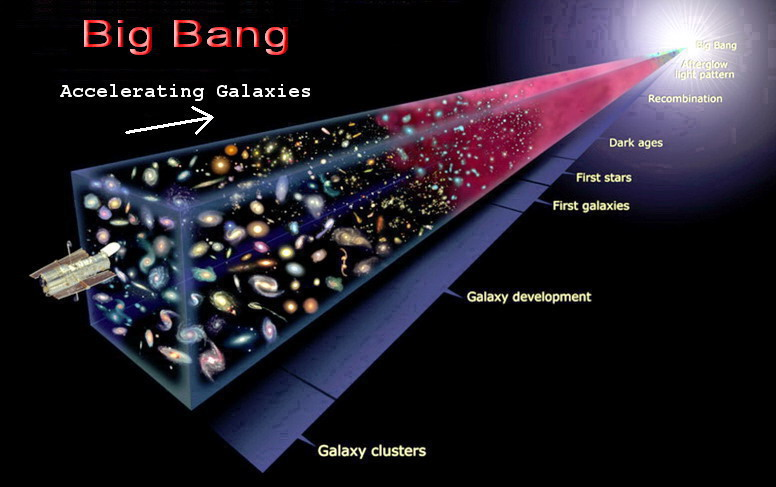According to the theory of Universe expansion, the younger the galaxy the faster it is moving away from us. That is, the acceleration of the galaxies occurs in the direction of the very first seconds after the Big Bang, and this acceleration is observed in any direction from us, indicating the absence of any logic in the theory. It turns out that the explosion is coming from the point of the Big Bang, and conversely, the acceleration occurs on any side of us. The proponents of this theory explain this absurdity by the fact that at the moment of the Big Bang all laws of physics and logic can be broken, and therefore there is "complete freedom of choice".

«At the point of the Big Bang and other singularities all the laws are violated therefore God keeps complete freedom of choice of what was happening in singularities and how the beginning of the Universe looked like.»

Also, the statement that the cosmic microwave background radiation is associated with the Big Bang, contradicts logic, as in this case it would have been observed on one side; on the side of the explosion, the radiation source. For example, if somewhere a star explodes, one point would be seen, the one where the explosion occurred. Radiation always comes from the point of radiation. It is applicable to all sources, even those that are more than 13 billion years old; they are also seen as points.

Due to the fact that on the basis of the theory of Universe expansion, the distance calculated using the Doppler effect is wrong, the distant galaxies appear to be small (compact), and type Ia supernovae have lower brightness. For example, galaxy UDFj-39546284, where z = 11.9, according to Hubble's quantum law, the distance is t = ln(z + 1)/H0 = 1.06·1018 sec = 33.8 billion light-years (10.4 Gpc), not 13.4 (4.1 Gpc), as considered in the expansion theory. That is why it seems compact.

«UDFj-39546284 is a compact galaxy, consisting of blue stars that have existed 13.4 billion years ago, that is, approximately 380 million years after the Big Bang. ... The galaxy has a redshift of z = 11.9.»
http://ru.wikipedia.org/wiki/UDFj-39546284 (In Russian)

According to the Big Bang theory, at z = 11.9 using the Doppler effect, the speed of the galaxy is close to the speed of light and time passes very slowly, all processes are slowed down z + 1 = 12.9 times. Accordingly, it turns out that we have aged 380 million years, and galaxy has aged 29.5 million years after the Big Bang. But the formation of galaxies requires about a billion years, and in such a short time the galaxy cannot be formed, and if we consider that by the Doppler effect the distance to distant galaxies is calculated incorrectly, time becomes negative.

«1 billion years - formation of the first galaxies.»
http://www.modcos.com/articles.php?id=105 (In Russian)

If vacuum field fluctuations exist, then in this vacuum there also would be electromagnetic wave energy absorption - a wave's energy transforms into fluctuation energy.

«At present it is ascertained that vacuum is not an "empty space". It is certain material continuum with quite determined, but unclear properties. It was confirmed by vacuum effects observation such as "zero-oscillations", vacuum polarization, particle generation by electromagnetic interactions. Therefore it is reasonable to suggest that physical vacuum could have real own internal "friction" resistance due to its own small but real viscosity. Thus, photons could interact with vacuum which could result in astronomical redshift of the light.»
http://bourabai.narod.ru/shtyrkov/evolution.htm (in Russian)

Waves are oscillations of a medium, as otherwise diffraction and interference would not been observed. During the oscillations there cannot be one hundred percent energy conversion, so the law of attenuation is effective for all waves, due to dissipation by conversion of one form of energy into another and back. No need to create myths, explaining the cosmological redshift by the intervention of dark energy, "wedging the galaxies off", since the usual formula of damped electromagnetic oscillations, taking into account the quantum principles, allows accurate calculation of the redshift for any distance.

«The chek-up showed that the data almost perfectly coincides with the model of "tired light" and strongly disagrees with Friedmann's model.»
http://www.vokrugsveta.ru/vs/article/6797/ (In Russian)
«Finally, after taking pictures of very distant objects (z of order 4) with the space telescope "Hubble", when it was expected to see the separate objects of the early Universe, close to the beginning of evolution, it became clear, that the complex structures (galaxies) are seen again. It is clear that it would take tremendous time for the formation of its structure, compatible with the age of the Universe calculated for the Big Bang model.»
http://bourabai.narod.ru/shtyrkov/evolution.htm (In Russian)
«... there is no significant evolution of the metallicity of the GRB host galaxies in the range 0 < z < 6.»
A report in the SAI 05.04.13. http://sed.sao.ru/~vo/cosmo_school/presentations/Sokolov_paper.pdf (In Russian)

One of the main tasks of the space telescope "Hubble" was to see the border of the Universe, but it has not been found. Everywhere there is roughly the same pattern of galaxies, which do not differ by age. The Universe is homogeneous, isotropic, there is no evolution of metallicity and no visible signs of the Big Bang.

Distance calculations by the method of "standard candles" showed that the cosmological redshift is in exact accordance with the formulas of Hubble's quantum law. In fact it turns out that the Nobel Prize in 2011 has been awarded for the confirmation of the Hubble's quantum law.

Distribution of the quantum principles to the astrophysics helped to conclude Hubble's quantum law, which, in contrast to the Doppler effect (the Big Bang theory) allows correct calculation of the cosmological redshift for any distance, as confirmed by the modern method of the "standard candles".

«Hubble's quantum law» in the «General Science Journal» (PDF)

Braking of the space crafts caused by the vacuum fluctuations